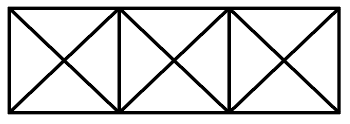Question 10

# How many triangles are there in the following figure ?Solution

As per the mentioned diagram,

Hence, total number of triangle = 12+12+4=28

• Free SSC Study Material - 18000 Questions
• 230+ SSC previous papers with solutions PDF